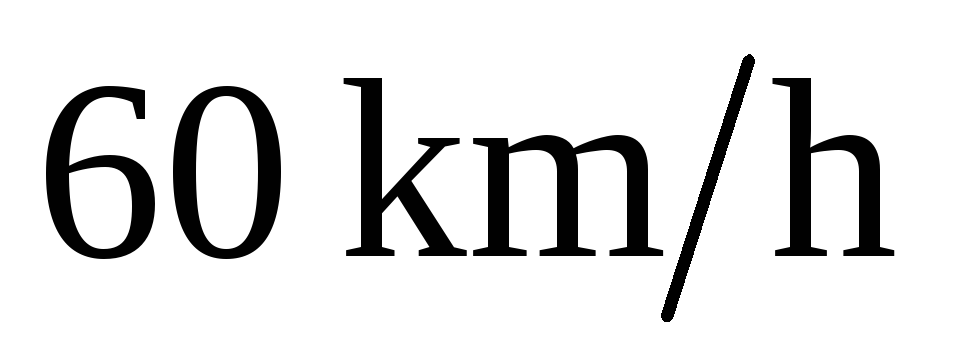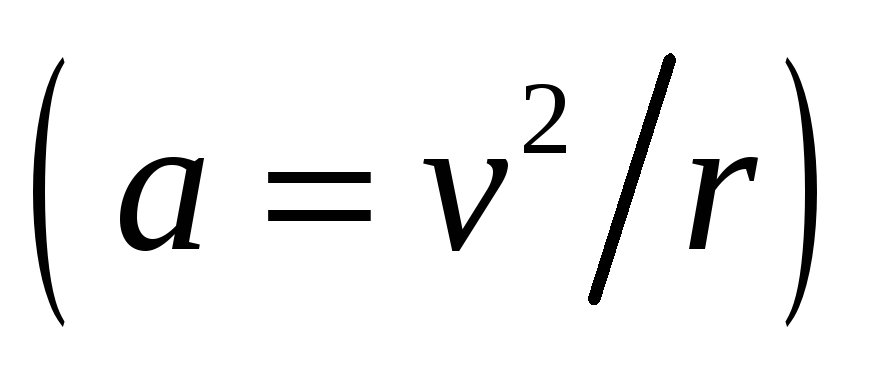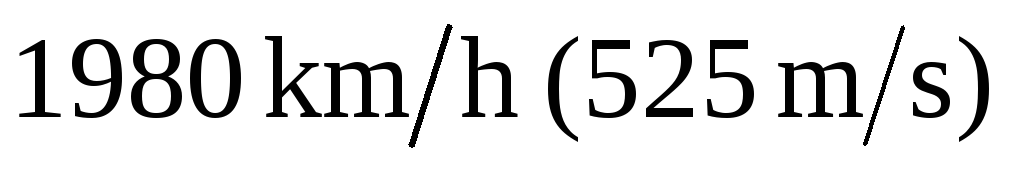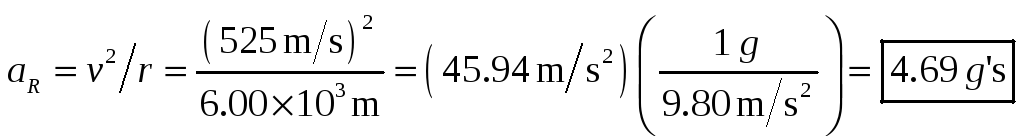# 5. 1 Kinematics of Uniform Circular Motion

 Honors Physics Homework Solutions “5.1 Kinematics of Uniform Circular Motion” page 129 Questions 2,6 2. Will the acceleration of a car be the same when the car travels around a sharp curve at a constantas when it travels around a gentle curve at the same speed? Explain. The centripetal acceleration for an object moving in circular motion is inversely proportional to the radius of the curve, given a constant speed. So for a gentle curve (which means a large radius), the acceleration is smaller, while for a sharp curve (which means a small radius), the acceleration is larger. 6. How many “accelerators” do you have in your car? There are at least three controls in the car which can be used to cause the car to accelerate. What are they? What accelerations do they produce? The three major “accelerators” are the accelerator pedal, the brake pedal, and the steering wheel. The accelerator pedal (or gas pedal) can be used to increase speed (by depressing the pedal) or to decrease speed in combination with friction (by releasing the pedal). The brake pedal can be used to decrease speed by depressing it. The steering wheel is used to change direction, which also is an acceleration. There are some other controls which could also be considered accelerators. The parking brake can be used to decrease speed by depressing it. The gear shift lever can be used to decrease speed by downshifting. If the car has a manual transmission, then the clutch can be used to decrease speed by depressing it (friction will slow the car). Finally, shutting the car off can be used to decrease its speed. Any change in speed or direction means that an object is accelerating. Problems 2,5,6 2. (I) A jet plane travelingpulls out of a dive by moving in an arc of radius 6.00 km. What is the plane’s acceleration in g’s? Find the centripetal acceleration from Eq. 5-1.Download 173.32 Kb.Share with your friends: